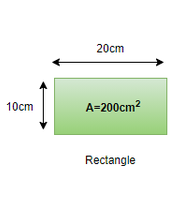# How to find the Area of a Rectangle?

• Last Updated : 06 Feb, 2022

Mensuration is a Greek term that denotes ‘measurement’. Mensuration is a department of arithmetic that concerns the analysis of geometric figures like squares, rectangles, cones, cylinders, etc. With the benefit of mensuration, one can understand how to compute the area, parameter, surface area, etc. which are the fundamentals of nearly every estimate in the area of Mathematics. Now, Let’s start with the most essential formula of the mensuration i.e. Area. Let’s preferably comprehend what an area is.

Area

The area is the size of a surface or the part of the area inhabited by a secure area. It is estimated for 2D sculptures and is represented in units like m2, cm2, etc. The unit of measurement is consistently a square team. It is indicated with the letter A.  The diagram down delivers examples of geometric figures along with their estimated area,Lets now examine the formula for the estimation of the area of the rectangle

What is the formula for the area of a square and rectangle?

The rectangle is a geometric figure with four flanks whose per angle is 90°. Out of the four flanks, the two flanks of the rectangle are similar and parallel with one flank longer than the other.

Area= L×B

Formula

The formula for computing the area of a rectangle can be emanated with the benefit of the down-mentioned steps:

1. The length and breadth (Width) of the rectangle should be understood in advancement.
2. Length and breadth are multiplied and the outcome acquired is the essential area.
3. The unit of area is square of the unit of its length and breadth

From the overhead steps, the formula of a rectangle can be reported like this.

Area of a rectangle (A) = Length(L) × Breadth(B)

where L is the length of the rectangle and B is the breadth of the rectangle.

Note: If the unit of length and breadth is not exact then it should be transformed into one unit. For e.g. If the length is in cm and breadth in m then both flanks should be adjusted either to m or cm.

Properties of a rectangle

1. The opposing flanks of the rectangle are similar and parallel.
2. The diagonal is of an identical length.
3. All the angles are 90°.
4. The diagonals of a rectangle bisect each other.

### Sample Problems

Problem 1: The length and width of a rectangle are 6 units and 3 units respectively. Find the area of the rectangle.

Solution:

Given,

length =6 unit

width=3 units

Area of rectangle= length x breath

= 6 × 3

=18 square units

Problem 2: Find the area of a rectangle whose height is 10 cm and width is 2 cm.

Solution:

Given, Height = 10 cm and Width = 2 cm

Area of a rectangle = width × height

Area of a rectangle = 10 × 2

Therefore, the area of the rectangle = 20 cm².

Problem 3: A rectangular panel has a width of 150 mm and a height of 99 mm. Find the area of this panel.

Solution:

Given, Height = 99 mm = 9.9 cm, and Width = 150 mm = 15 cm

Area of a rectangle = width × height

Area of a rectangle = 15 × 9.9

Therefore, the area of the rectangle = 148.5 cm².

Problem 4: The height of a rectangular net is seen to be 20 cm. Its area is seen to be 260 cm². Find the width of the provided net.

Solution:

Given, Height = 20 cm and Area = 260 cm2.

Area of a rectangle = width × height

Therefore, Width = Area / length

Width = 260/20

Width = 130 cm

Problem 5: The height and width of a rectangular desk are 40 m and 20 m respectively. If a carpenter charges ₹ 2 per m² for making the drawer, how much would it cost to make the whole desk?

Solution:

Given, Height = 40 m and Width = 20 m

Area of a rectangle = width × height

Area of a rectangle = 40 × 20

Area of a rectangle = 800 m²

At the cost of ₹ 2 per m², the cost for making desk 800 m² is 800 × 2 = ₹ 1600

Problem 6: A  wall whose length and width are 60 m and 40 m respectively needs to be painted. The quantity of the selected wall paint box is ₹ 2 per m². Find the total number of paint boxes needed to fully cover the wall.

Solution:

Given, Length = 60 m and Width = 40 m

Area of a rectangle = width × length

Area of a rectangle = 60 × 40

Area of the rectangle = 2400 m²

The number of paint boxes required for covering the wall can be counted by

Area of wall / Area of a paint box

2400/2 = 1200 tiles.

My Personal Notes arrow_drop_up